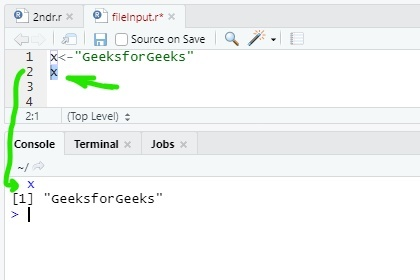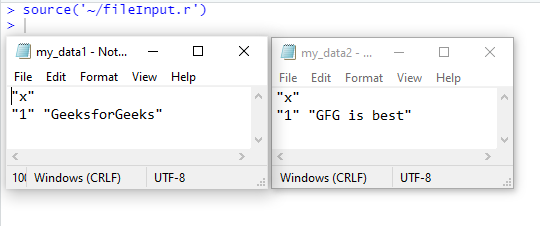# Printing Output of an R Program

In R there are various methods to print the output. Most common method to print output in R program, there is a function called `print()` is used. Also if the program of R is written over the console line by line then the output is printed normally, no need to use any function for print that output. To do this just select the output variable and press run button.
Example:

 `# select 'x' and then press 'run' button ` `# it will print 'GeeksforGeeks' on the console ` `x <- ``"GeeksforGeeks"` `x  `

Output:

` "GeeksforGeeks"`#### Print output using `print()` function

Using `print()` function to print output is the most common method in R. Implementation of this method is very simple.

Syntax:
print(“any string”)
or,
print(variable)

Example:

 `# R program to illustrate ` `# printing output of an R program ` ` `  `# print string ` `print``(``"GFG"``) ` ` `  `# print variable ` `# it will print 'GeeksforGeeks' on the console ` `x <- ``"GeeksforGeeks"` `print``(x)  `

Output:

``` "GFG"
 "GeeksforGeeks"
```

#### Print output using `paste()` function inside `print()` function

R provides a method `paste()` to print output with string and variable together. This method defined inside the `print()` function. `paste()` converts its arguments to character strings. One can also use `paste0()` method.

Note: The difference between `paste()` and `paste0()` is that the argument sep by default is ” “(paste) and “”(paste0).

Syntax:
print(paste(“any string”, variable))
or,
print(paste0(variable, “any string”))

Example:

 `# R program to illustrate ` `# printing output of an R program ` ` `  `x <- ``"GeeksforGeeks"` ` `  `# using paste inside print() ` `print``(``paste``(x, ``"is best (paste inside print())"``))  ` ` `  `# using paste0 inside print() ` `print``(``paste0``(x, ``"is best (paste0 inside print())"``))  `

Output:

``` "GeeksforGeeks is best (paste inside print())"
 "GeeksforGeeksis best (paste0 inside print())"
```

#### Print output using `sprintf()` function

`sprintf()` is basically a C library function. This function is use to print string as C language. This is working as a wrapper function to print values and strings togather like C language. This function returns a character vector containing a formatted combination of string and variable to be printed.

Syntax:
sprintf(“any string %d”, variable)
or,
sprintf(“any string %s”, variable)
or,
sprintf(“any string %f”, variable))
etc.

Example:

 `# R program to illustrate ` `# printing output of an R program ` ` `  `x = ``"GeeksforGeeks"` `# string ` `x1 = 255            ``# integer ` `x2 = 23.14          ``# float  ` ` `  `# string print ` `sprintf``(``"%s is best"``, x) ` ` `  `# integer print ` `sprintf``(``"%d is integer"``, x1) ` ` `  `# float print ` `sprintf``(``"%f is float"``, x2) `

Output:

```> sprintf("%s is best", x)
 "GeeksforGeeks is best"
> sprintf("%d is integer", x1)
 "255 is integer"
> sprintf("%f is float", x2)
 "23.140000 is float"```

#### Print output using `cat()` function

Another way to print output in R is using of `cat()` function. It’s same as `print()` function. `cat()` converts its arguments to character strings. This is useful for printing output in user defined functions.

Syntax:
cat(“any string”)
or,
cat(“any string”, variable)

Example:

 `# R program to illustrate ` `# printing output of an R program ` ` `  `# print string with variable ` `# "\n" for new line ` `x = ``"GeeksforGeeks"` `cat``(x, ``"is best\n"``)  ` ` `  `# print normal string ` `cat``(``"This is R language"``) `

Output:

```GeeksforGeeks is best
This is R language
```

#### Print output using `message()` function

Another way to print something in R by using `message()` function. This is not used for print output but its use for showing simple diagnostic messages which are no warnings or errors in the program. But it can be used for normal uses for printing output.

Syntax:
message(“any string”)
or,
message(“any string”, variable)

Example:

 `# R program to illustrate ` `# printing output of an R program ` ` `  `x = ``"GeeksforGeeks"` `# print string with variable ` `message``(x, ``"is best"``)  ` ` `  `# print normal string ` `message``(``"This is R language"``) `

Output:

```GeeksforGeeks is best
This is R language ```

#### Write output to a file

To print or write a file with a value of a variable there is a function called `write()`. This function is used a option called table to write a file.

Syntax:
write.table(variable, file = “file1.txt”)
or,
write.table(“any string”, file = “file1.txt”)

Example:

 `# R program to illustrate ` `# printing output of an R program ` ` `  `x = ``"GeeksforGeeks"` `# write variable  ` `write.table``(x, file = ``"my_data1.txt"``) ` ` `  `# write normal string ` `write.table``(``"GFG is best"``, file = ``"my_data2.txt"``) `

Output:My Personal Notes arrow_drop_up3rd year student of Information Technology JADAVPUR UNIVERSITY

If you like GeeksforGeeks and would like to contribute, you can also write an article using contribute.geeksforgeeks.org or mail your article to contribute@geeksforgeeks.org. See your article appearing on the GeeksforGeeks main page and help other Geeks.

Please Improve this article if you find anything incorrect by clicking on the "Improve Article" button below.

Article Tags :

1

Please write to us at contribute@geeksforgeeks.org to report any issue with the above content.